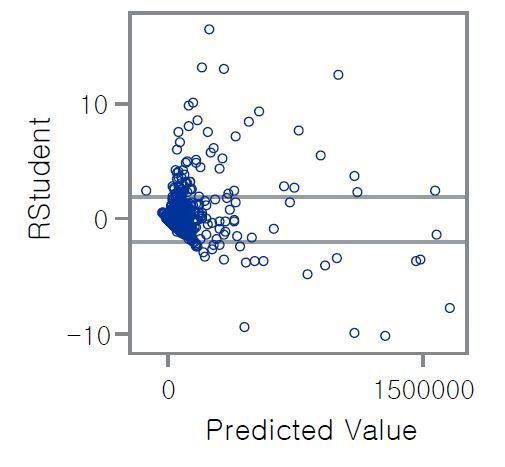## Is It possible to delete outlier observation under rstudent condition in reg procedure ?

Hello . Thank you for your time.

I have some terrible question.

I have to clear the observation that out of (-2,2) rstudent .Does anybody know how to delete those observation out of (-2,2) ,by coding ? there are too many to find and delete manually...

1 ACCEPTED SOLUTION

Accepted SolutionsKsharp
Super User

## Re: Is It possible to delete outlier observation under rstudent condition in reg procedure ?

Use OUTPUT statement to save rstudent:

``````proc reg data=sashelp.class;
model weight=age height;
output out=want rstudent=rstudent cookd=cookd;
quit;``````

and proc sort it ,delete obs you don't want.

4 REPLIES 4

## Re: Is It possible to delete outlier observation under rstudent condition in reg procedure ?

From you graph it appears that you may have a variable rstudent with values of concern. If that is the case then what you want is fairly simple:

Data want;

set have;

where -2 le rstudent le 2;

run;

## Re: Is It possible to delete outlier observation under rstudent condition in reg procedure ?

Thank you for your reply . but This graph is part of the proc reg result .

for example :

proc reg data = work.aa;

model y=x1 x2 x3;

run;

so i don't have rstudent variable .

what i want to know is how can i make these variable ?Ksharp
Super User

## Re: Is It possible to delete outlier observation under rstudent condition in reg procedure ?

Use OUTPUT statement to save rstudent:

``````proc reg data=sashelp.class;
model weight=age height;
output out=want rstudent=rstudent cookd=cookd;
quit;``````

and proc sort it ,delete obs you don't want.

## Re: Is It possible to delete outlier observation under rstudent condition in reg procedure ?

You can use dataset options on output data sets in procedures that create data sets directly:

```proc reg data=sashelp.class;
model weight=age height;
output out=want (where=( 2 le rstudent le 2))
rstudent=rstudent cookd=cookd;
quit;```
Discussion stats
• 4 replies
• 1510 views
• 1 like
• 3 in conversation